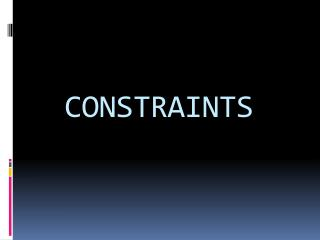DownloadDownload PresentationCONSTRAINTS

# CONSTRAINTS

Download Presentation## CONSTRAINTS

- - - - - - - - - - - - - - - - - - - - - - - - - - - E N D - - - - - - - - - - - - - - - - - - - - - - - - - - -
##### Presentation Transcript

1. CONSTRAINTS

2. TOPICS TO BE DISCUSSED • DEFINITION OF CONSTRAINTS • EXAMPLES OF CONSTRAINTS • TYPES OF CONSTRAINTS WITH EXAMPLES

3. CONSTRAINTS In order to solve a set of differential equations for the motion of a system of n-particles, we have to impose certain restrictions on the positions and velocities of the particles of the system. Such geometrical or kinematical restrictions on the motion of a particle or system of particles are called constraints. EXAMPLES OF CONSTRAINED MOTION • The motion of a rigid body restricted by the condition that the distance between any of its two particles remains unchanged. • The beads of an abacus constrained to one dimensional motion by the supporting wires. • Motion of the gas molecules within a container restricted by the walls of the vessel.

4. TYPES OF CONSTRAINTS • HOLONOMIC AND NON HOLONOMIC • SCLERONOMIC AND RHEONOMIC

5. Holonomic Constraints: For a constraint to be holonomic its conditions must be expressible as equations connecting the coordinates of the particles (and , if possible, time also) i.e. in the form of equation : F(r1 ,r2,………,rn, t)=0 Where r 1,r2,………..,rnrepresent the position vectors of the particles of a system and t the time. • Hence, a holonomic constraint depends only on • the coordinates and time . • It does not depend on the velocities.

6. EXAMPLES OF HOLONOMIC CONSTRAINTS • CONSTRAINTS INVOLVED IN THE MOTION OF RIGID BODIES: l ri – rjI2= Cij2 where Cij are constants and ri and rj are the position vectors of ith and jth particles.

7. Constraints involved in the motion of the point mass of simple pendulum | r– a | = l2

8. If the conditions of the constraints cannot be expressed as equations connecting the coordinates of particles of the system, they are called non-holonomic constraints. The conditions of these constraints are expressed in the form of inequalities. NON-HOLONOMIC CONSTRAINTS

9. Examples of non-holonomic constraints • constraints involved in the motion of a particle placed on the surface of solid sphere i.e. • r2 –a2 >0 where a is the radius of the sphere. • constraint on an object rolling on a rough surface without slipping. • constraints involved in the motion of gas molecules in a container i.e. • r3 <= a3

10. The constraints which are independent of time are called scleronomic constraints e.g. a bead sliding on a rigid curved wire fixed in space SCLERONOMIC CONSTRAINTS:

11. The constraints which contain time explicitly are called rheonomic constraints. e.g. 1) a bead sliding on a rigid curve wire moving in some prescribed fashion. 2)if we construct a simple pendulum whose length changes with time i.e. l=l(t) then the constraints expressed by the equations are time dependent, hence, rheonomic . RHEoNOMIC CONSTRAINTS

12. An example to illustrate the difference between holonomic and non- holonomic constraints The motion of a particle constrained to lie on the surface of a sphere is subject to a holonomic constraint. But if the particle is able to fall off the sphere under the influence of gravity, the constraint becomes non-holonomic.

13. THANX